# How to Use the PERCENTILE Function in Excel

Joshua earned an MBA from USF and he writes mostly about software and technology.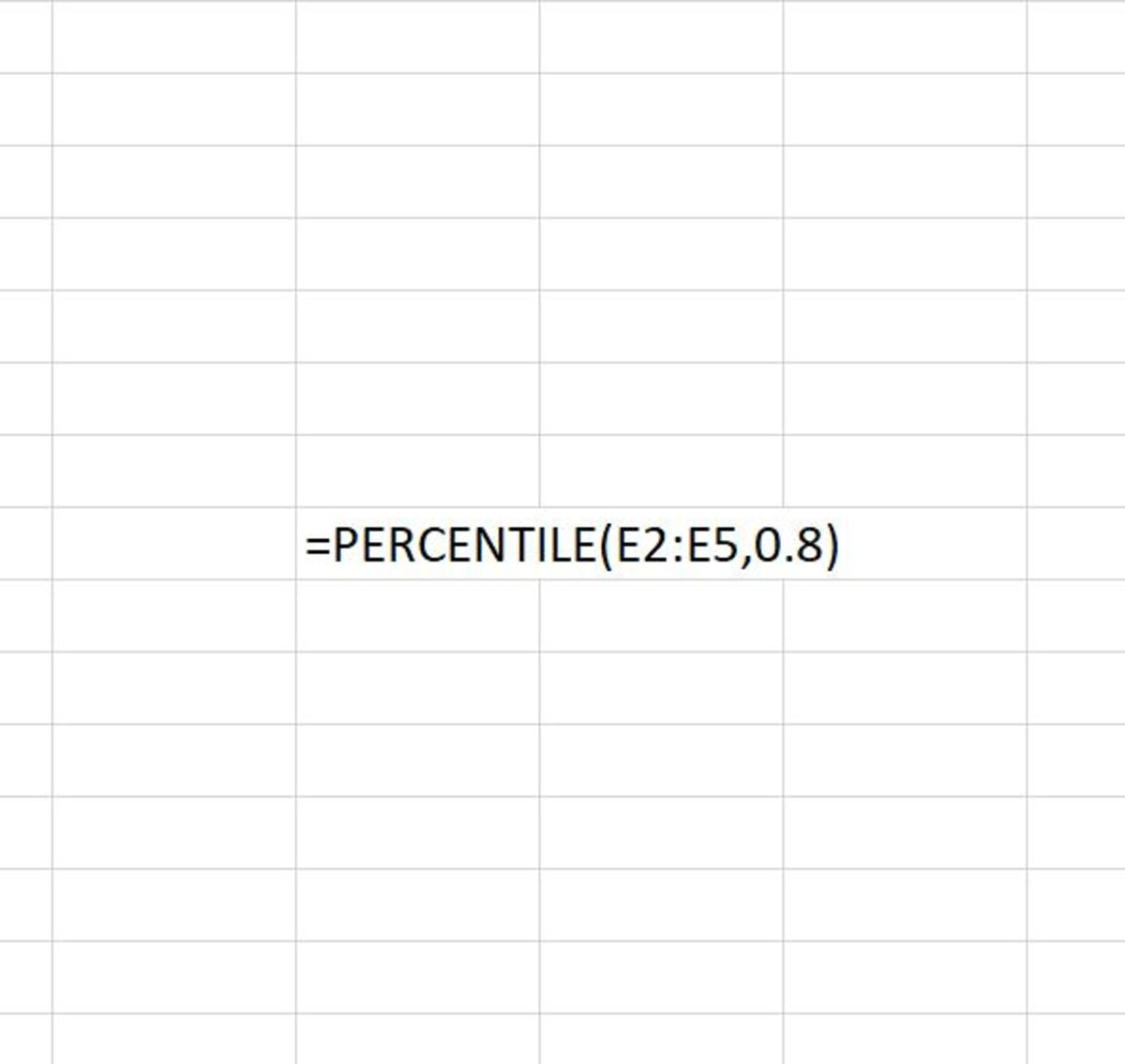This illustration from Excel above shows the PERCENTILE function being used in Excel to find the 80th percentile of the cell range E2:E5. By default, the 80th percentile would be included in the results. Created by Joshua Crowder

## The Purpose of the PERCENTILE Function

The PERCENTILE function takes data from a range and returns the kth percentile. This function allows you to select a range and what percentile you want to be returned. Examples of percentiles returned would include the 90th, 50th, or even 10th percentile of a range of numbers.

Just for a refresh on what a statistical percentile is, if you tested in the 25th percentile of your class, this would mean that 25% of your class tested lower than you did.

## How It Works

When inputted the PERCENTILE function must be entered like a formula. To add this function you would complete the following steps:

1. Click in the cell where the result is to display.
2. Type "=PERCENTILE(". Without the quotations marks of course!
3. The array of numbers is chosen followed by a comma. This is the range of numbers that you are finding the percentile for.
4. The percentile that needs to be returned is followed by a closing parenthesis. This number should be entered in the form of a decimal.
5. Lastly, the data need to be entered into the cell.

The syntax for the PERCENTILE function can be seen in more detail here:

=PERCENTILE(array,k)

There are two arguments in this function, the array, and k. The array defines the data set and k dictates what percentile value is chosen from zero to one. Both of these arguments are required for this function to work.

The array can be entered as an array or a named range.

## Inserting the PERCENTILE Function

The PERCENTILE function can be added to a cell with the use of an inserting method that Excel provides. If you have problems remembering how to use the syntax for this function, inserting it is the best option.

To use this method, a cell must first be selected. Next, the formulas tab needs to be selected, followed by clicking on the "More Functions" button on the Excel ribbon. Compatibility should be chosen along with the PERCENTILE selection from the list.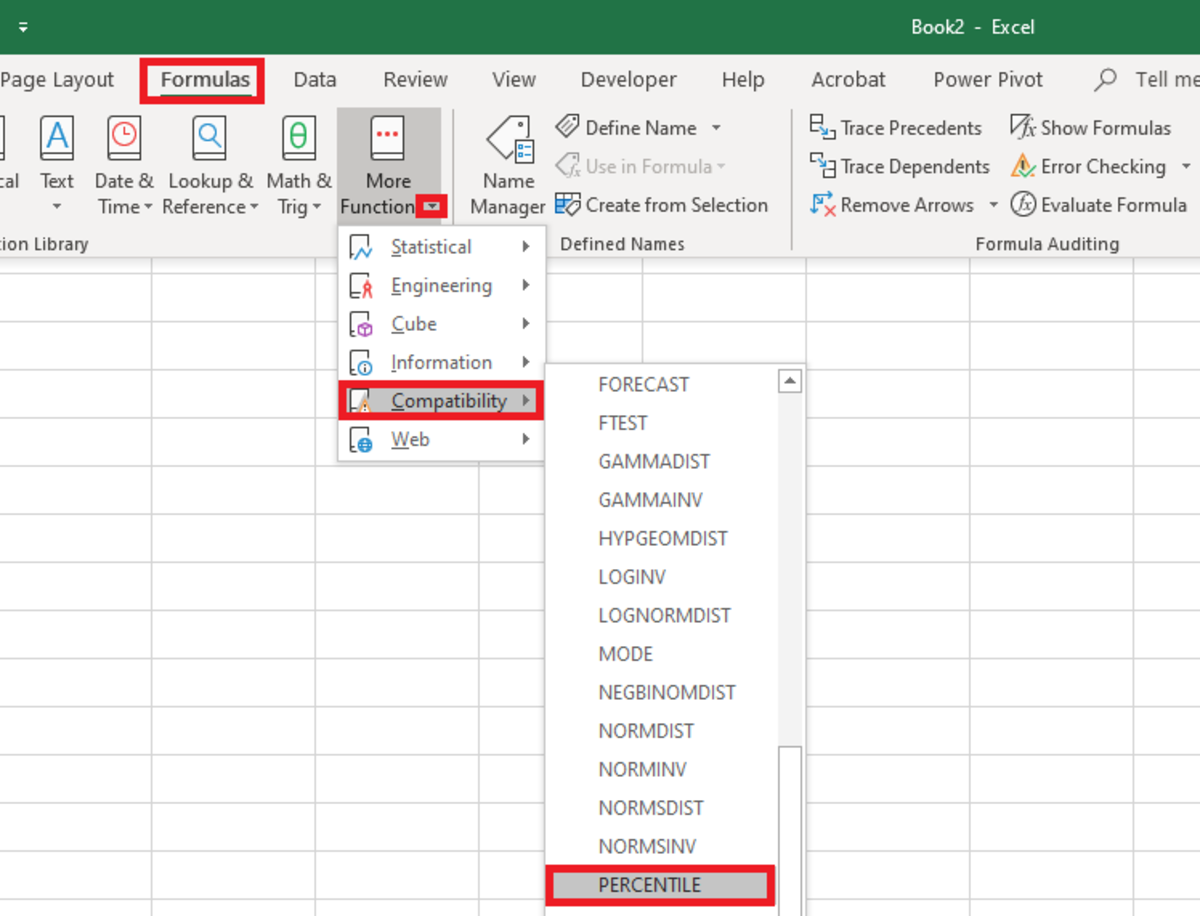To insert the PERCENTILE function from the formula tab you must first navigate to Formulas→More Functions→Compatibility→PERCENTILECreated by Joshua Crowder

Next, a functions argument window appears where the array of data and kth percent needs to be added.

The array can be typed in or it can be selected from the spreadsheet by clicking the up arrow to the right of the array field. The kth percentile can be typed in decimal form or a cell reference can be chosen by clicking on the arrow to the right of that field.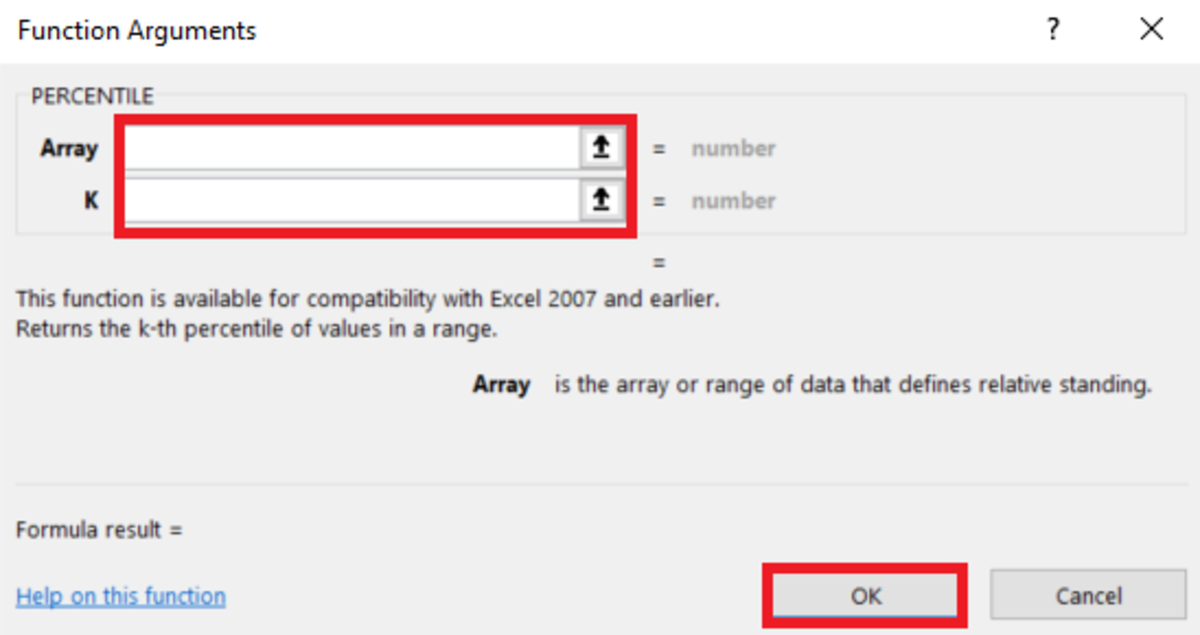Here in the functions argument window an array and k can be selected for the function. Created by Joshua Crowder
Scroll to Continue

## PERCENTILE Function Syntax Examples

Below are some examples of the PERCENTILE function being used in varying situations.

=PERCENTILE(range1,.4) - 40th percentile

• Here the function uses a named range as an input for an array and the k chosen is .4. As a result, the 40th percentile of the named range range1 will display.

=PERCENTILE(range1,D1)

• Here the function uses a named range as an input for an array and the k chosen is D1. As a result, the percentile of range1 will display depending on the k that appears in cell D1.

=PERCENTILE(range1,80%)

• 5the function uses a named range as an input for an array and the k chosen is 80%. As a result, the percentile of the named range range1 will display the 80th percentile.

=PERCENTILE(C5:C14.,E5)

• In this final syntax example, the reference range C5:C14 serves as the array and the k is located in the cell reference E5. The percentile of the referenced range C5:C14 will display depending on the k that appears in cell E5.

As you can see from the above examples, there are a few different ways that the arguments can be displayed in the function.

## Practical Example

In this section, I will walk you through a complete example of inserting the PERCENTILE function where the function is searched from a list of functions. The 20th percentile of a dataset will be found. See the simple data set in the illustration below.

To use the insert function a cell needs to be clicked and the formulas tab needs to be clicked.

Next, the insert function can be clicked. Type percentile in the search bar that appears and select percentile from the list.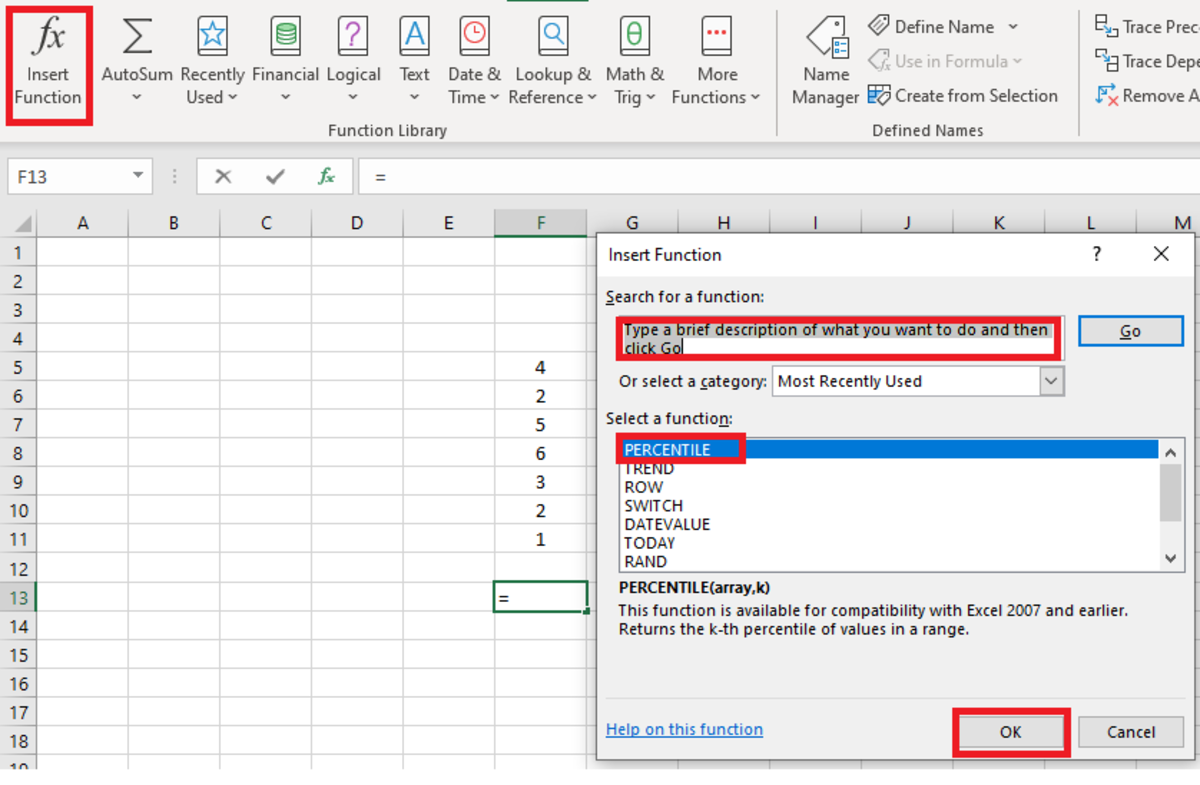If you are unsure which category a function is located under, a function can always be searched for by clicking on the insert function button. Created by Joshua Crowder

Next, the range that you want to find the percentile of needs to be selected after clicking in the array field. This is followed by adding a number between .1 and 1 after clicking the field labeled k.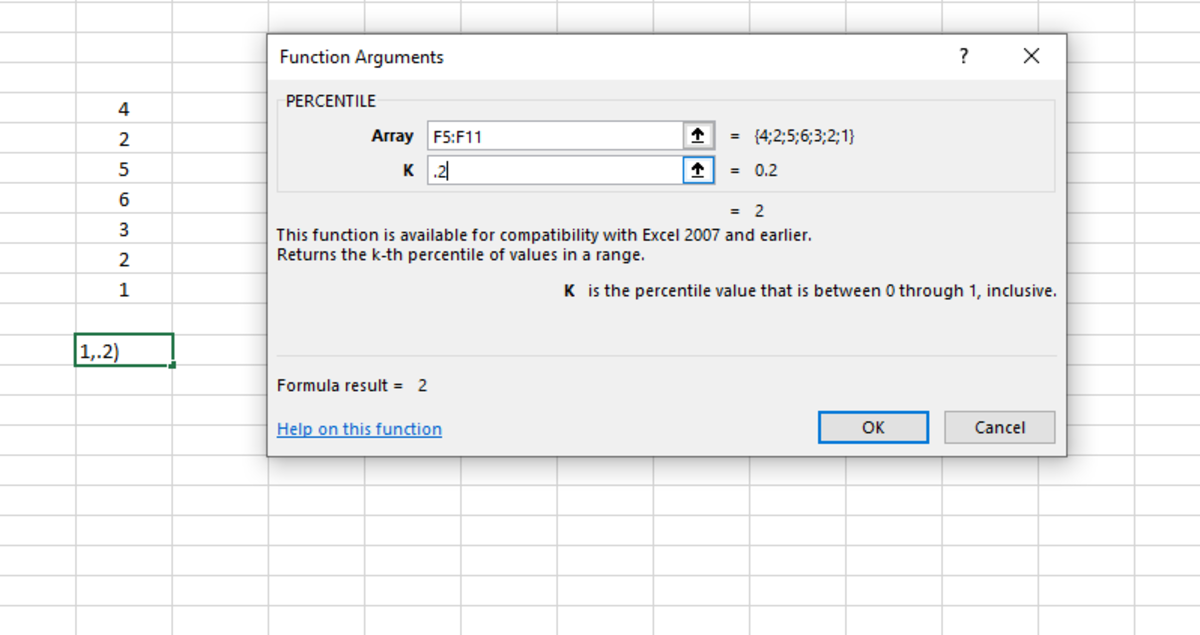When selecting k remember that the a decimal or a percentage under 1 or 100% can be chosen. Created by Joshua Crowder

After the OK button is selected, the 20th percentile of the data set appears as a result.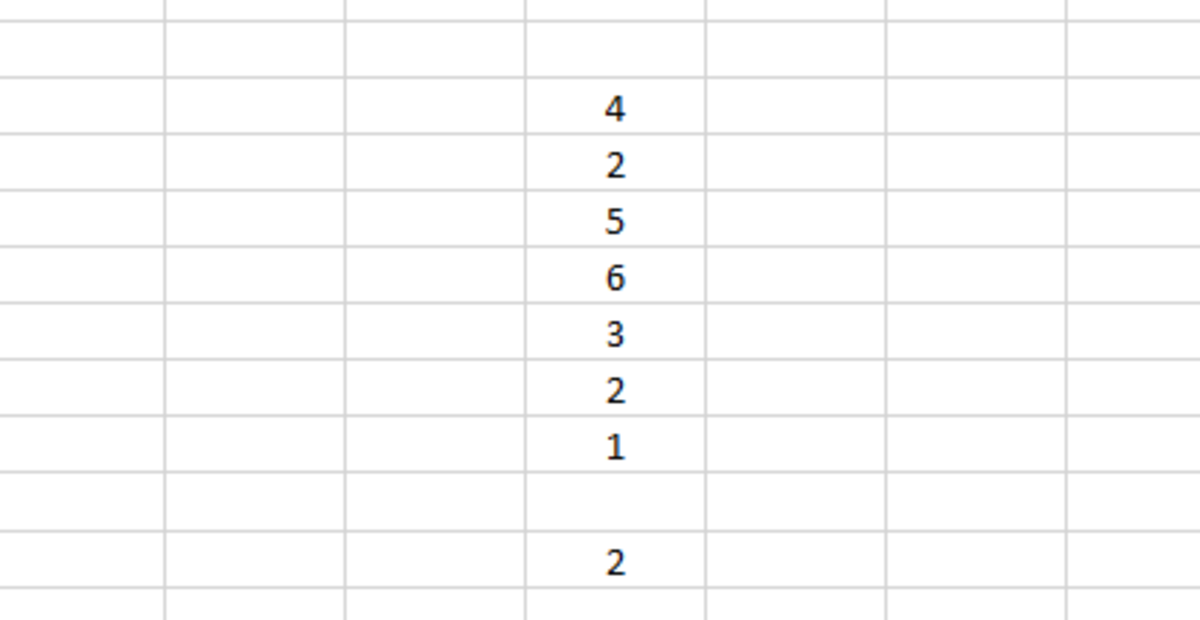Here the 20th percentile of this data set is 2.Created by Joshua Crowder

This means that 2 is where 20% of the data is found. So, 2 is in the 20th percentile of the data set.

## Similar Excel Functions

Two other functions are used to find percentiles. More recently Microsoft has created new percentile functions that are specific in regards to including and excluding the percentile in the results.

Each of these functions is briefly described below.

PERCENTILE.EXC - This shows the percentile excluding the percentile chosen in the results.

PERCENTILE.INC - This function will produce the same result as the Percentile function because they both include the chosen percentile in the results.

Microsoft. (n.d.). PERCENTILE function. Retrieved January 5, 2020, from https://support.office.com/en-us/article/percentile-function-91b43a53-543c-4708-93de-d626debdddca.

## Learning More About Excel Functions

To learn more about using functions in Excel I recommend purchasing Excel Formulas & Functions For Dummies.

This content is accurate and true to the best of the author’s knowledge and is not meant to substitute for formal and individualized advice from a qualified professional.

© 2020 Joshua Crowder